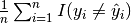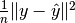# Model Tuning¶

This part of the package provides a simple API for model-tuning routines.

gen_cross_validate(evalfun, n, model)

Perform in parallel a generic cross-validation (CV) routine defined in evalfun by the splitting specified in model.cv_gen.

Parameters: evalfun – function to evaluate n – total number of data points (instances) to create Kfold CV generator if model.cv_gen is undefined (null) model – SALSAModel which contains the cv_gen field of type Nullable{CrossValGenerator}  or model.output.cv_folds field containing predefined indices for each fold an average of evalfun evaluations.
misclass(y, yhat)

Calculate misclassification rate as.

mse(y, yhat)

Calculate mean squared error asauc(y, yhat[, n=100])

Calculate Area Under ROC Curve. Default number of thresholds is 100.

Footnotes

  wrapper around the type defined in MLBase.jl package